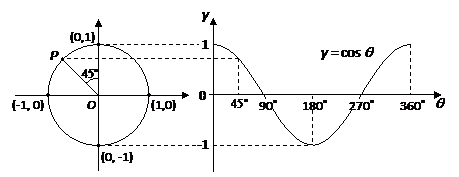top of page
Search
• Stu WØSTU

# Working with Cosine in AC Power Calculations

When AC voltage and current waveforms are not in phase with one another, the power delivered to a circuit will be only a portion of the power computed by multiplying voltage (E) and current (I). The fraction of powered delivered to the circuit is determined by the phase angle between voltage and current waveforms. The delivered power is called the real power.

Real power is computed as:

P(real) = EI cos Θ

where Θ (theta) is the phase angle between voltage and current waveforms.

The value of cos Θ will be between 0 and 1, and this fractional value is called the power factor. Power Factor (PF) = cos Θ.

To determine the real power, simply complete the multiplication of the three terms: voltage x current x cosine Θ. Voltage and current values are trivial, but let's see how to get a value for cosine Θ, or power factor, using a common scientific calculator.

Mathematically, cosine values vary between -1 and 1 depending on the angle. Some cosine and angle values are:Notice the cyclical nature of the cosine values. They vary between -1 and 1 as the angle cycles through 360 degrees. The cosine function is determined by the vertical, or y axis value, of a point on a circle as the interior angle of the circle rotates about the 360-degrees of the circle, as illustrated in this graph:Cosine values are easily obtained with a scientific calculator as follows:

1. Make sure the calculator mode is set to degrees. (Not radians.)

2. Enter the angle in degrees.

3. Select the trigonometry function "cos" for cosine.

Suppose we want to compute the power factor for an RL circuit that produces a 45-degree phase angle between voltage and current. The following video illustrates calculation of cosine using a scientific calculator. Cosine 45 degrees = 0.707 (rounded). (PF = 0.707 for a 45-degree phase angle between E and I.)

67 views

See All
bottom of page